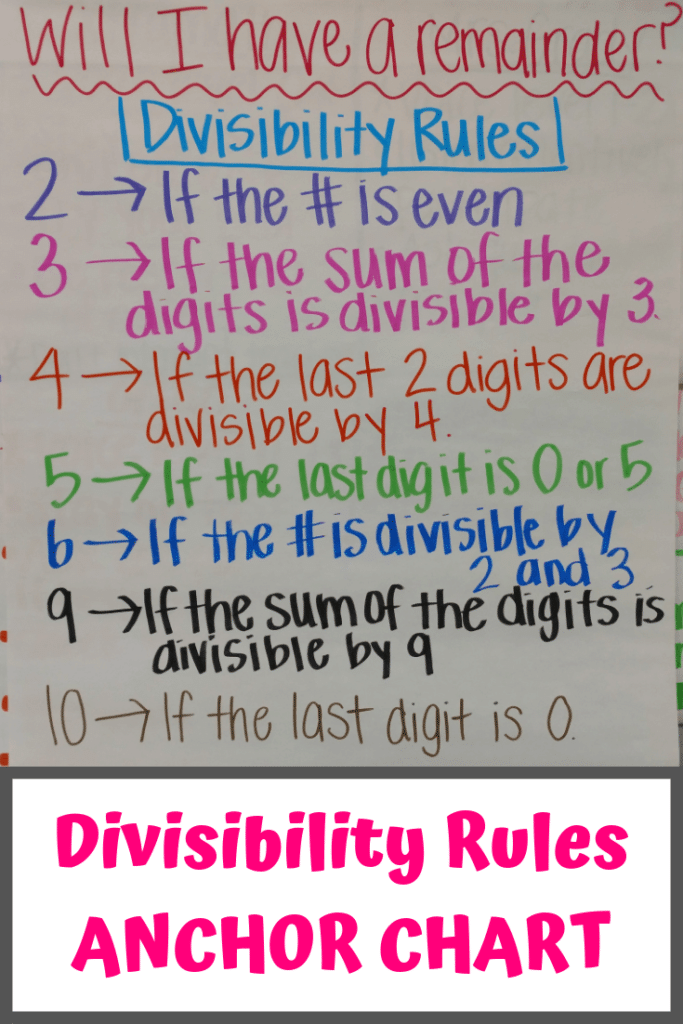# Divisibility Rules Anchor Chart for Fifth Grade Math

When teaching division to my fifth graders, I have found that having a divisibility rules anchor chart in my classroom is very helpful!  Division and decimals seem to be two concepts that are difficult for my fifth-grade math students to understand.

When I can get my students to remember these divisibility rules, they do significantly better at division.  For instance, I encourage them to use these rules as a way to double-check their work when doing long division.  If, after dividing, they end up with a remainder when these rules say there should be none, then they need to go back and check their division.

## My Divisibility Rules Anchor Chart

There are divisibility rules for most numbers, but I selected the rules that I felt were most appropriate for their level.  After all, they are only fifth graders and are somewhat new to this concept.  No need to remember 30 rules for the first 30 numbers!

For my divisibility anchor chart, I picked some rules numbers like 2, 5, and 10 that are easy to remember and very intuitive. Gotta give the students some confidence!

Other numbers like 3, 4, 6, and 9 require more thought.  Their divisibility rules will require a little bit more memorization at first before the students understand the proof of concept.

In the beginning, the students refer to the anchor chart a lot, but after repetition and practice, these rules become ingrained.  They also begin to understand a little more the reason why certain numbers don’t have a remainder when doing long division.## The Rules I’ve Written – Will I Have a Remainder?

Here are the divisibility rules on my math anchor chart.

• 2: A number is divisible by 2 if it is even.
• 3: A number is divisible by 3 if the sum of all the digits is divisible by 3.
• 4: If the last 2 digits are divisible by 4, then the number itself is divisible by 4.
• 5: A number is divisible by 5 if the last digit is a 0 or 5.
• 6: If the number is divisible by 2 and 3, then it is also divisible by 6.  (This rule makes a lot of sense to the students actually!)
• 9: If the sum of the digits is divisible by 9, so is the number.
• 10: A number is divisible by 10 if the last digit is a 0.